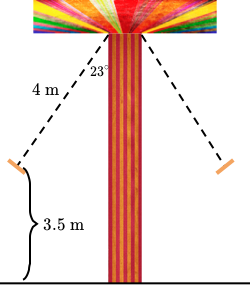## General Right Triangle Word Problems

Howard is designing a chair swing ride. The swing ropes are 4 meters long, and in full swing, they tilt in an angle of 23°. Howard wants the chairs to be 3.5 meters above the ground in full swing.

How tall should the pole of the swing ride be?In order to do this problem, we have to apply the law of sines.

sin (67°) / x = sin (90°) / 4
x = 3.68

Where does 67° come from?
If we draw a line perpendicular to the pole to the hypotenuse, we got a 90° angle. So, 180°- (90° + 23°) = 67°.

We haven’t finished yet, we need to add x to 3.5 because they ask the entire length of the pole.
3.68 m + 3.5 m = 7.18 m

How tall should the pole of the swing ride be?

## Exponential Vs Linear Growth over time

Aril and Dita are wealthy Norwegian business owners who make monthly donations to international disaster relief.

• Aril donated 150 Norwegian kroner  the first month and the cumulative number of Norwegian kroner she has donated increases by a factor of 2.5 each month.
• Dita donated 300 KR the first month and the cumulative number of Norwegian kroner she has donated increases by 400 KR each month.
They started making donations at the same time, and they both make their monthly donations at the beginning of each month.

What is the first month in which Aril’s cumulative donation exceeds Dita’s cumulative donation?

How do we go about solving this problem? First, let’s identify which statement describes exponential and which statement describes linear growth.

Exponential growth is a growth whose rate is rapid in proportion to the function’s current value. Linear growth is a growth whose rate is constant over time.

Based on the context, we can say that Aril’s donation is an exponential growth and can be model by an equation:

y = 150 * (2.5)^(x-1)

And Dita’s donation is a linear growth and can be modeled as:

y = 300 + 400(x-1)

Why (x-1)? Because in the first month which is x, Aril and Dita have already donated 150 KR and 300 KR respectively. Therefore, we have to subtract the first month to know the rate of the following months.

Now, let’s figure out which month Aril’s donation exceeds Dita’s donation. Let’s think of it graphically.

We know that exponential growth started off slowly and will eventually outreach linear growth; therefore, we have to look for its intersection.

The two lines intersect at 3.28, 1212.17. Therefore, we can conclude that at month 4, Aril’s donation will exceed Dita’s.

What is the first month in which Aril’s cumulative donation exceeds Dita’s cumulative donation?

## Inequalities

For past weeks, my math class has been working on inequalities. We have been focusing on this lesson for quite a few weeks. So, an inequality is a statement which indicates that one quantity is greater than or less than another. We can write the statement using symbols such as “<“, “>”, “≥”, and “≤”. But, this concept has gotten deeper rather than just 1 < 2. However, we have done lots of activities associated with inequalities such as Inequalities word problem, Two-step inequalities, compound inequalities; furthermore, we also looked at inequalities systems and graphs which includes Graphs of Inequalities, Systems of inequalities graphs…etc. On the other hand, we’re not just learning in a textbook, but all of these activities come from Khanacademy.org website which allows us to practice lots of math in an efficient way.